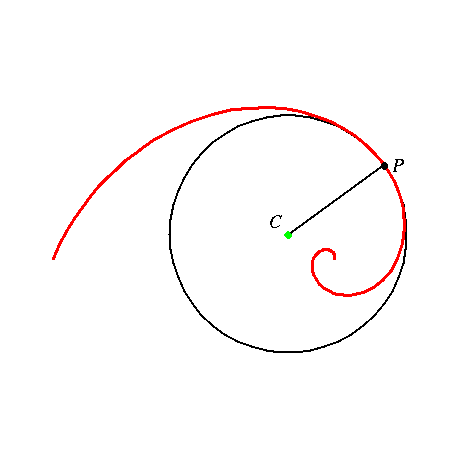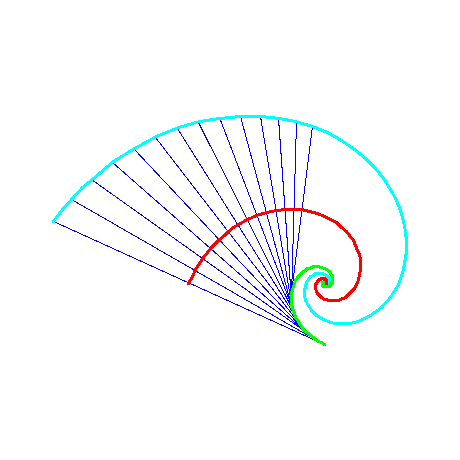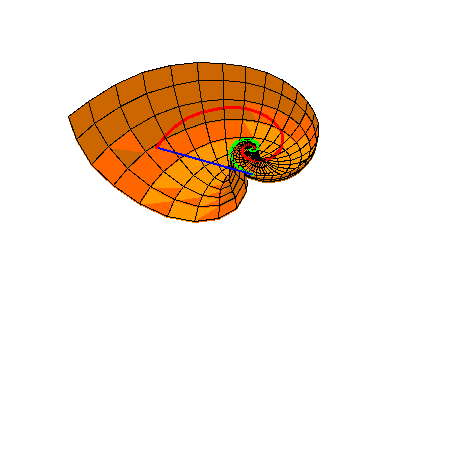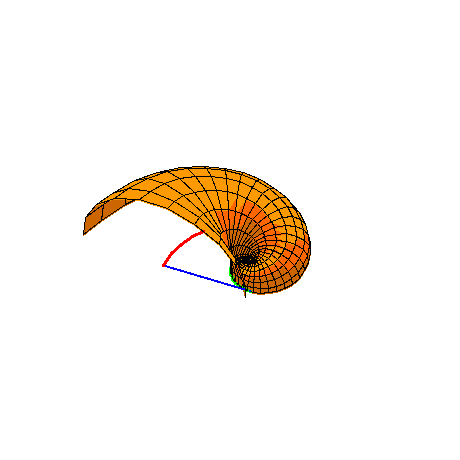# MATHEMATICS OF A NAUTILUS SHELL

Figure 1

#### At each point P of a plane curve, in particular of a logarithmic spiral, there is a well defined circle that among all circles is the best possible approximation to the curve: the so-called circle of curvature, with center C in the center of curvature, and the radius of curvature as radius. The construction is depicted in Figure 2.Figure 2

#### The centers of curvature make a curve, which is called the evolute of the curve; the green curve in Figure 3. For the logarithmic spiral, the evolute is again a logarithmic spiral. It can be constructed by rotating the logarithmic spiral through a right angle and multiplying by cotangent of u. Symmetrical to the evolute with respect to the logaritmic spiral, there is another logaritmic spiral, which we call the exterior evolute. The exterior evolute is depicted in Figure 3 together with a number of ribbons connecting corresponding points on the evolute and the exterior evolute.Figure 3

#### For exactly one angle u, approximately 69.5 degrees, the evolute and the exterior evolute of the logarithmic spiral fit each others; cf. Figure 4. In the following we use this unique angle in the logarithmic spiral.Figure 4

Figure 5

Figure 6

Figure 7

#### The bottom half and the top half of the Nautilus shell is shown in respectively Figure 8 and Figure 9.Figure 8Figure 9